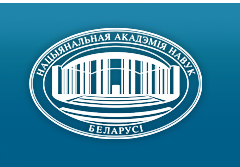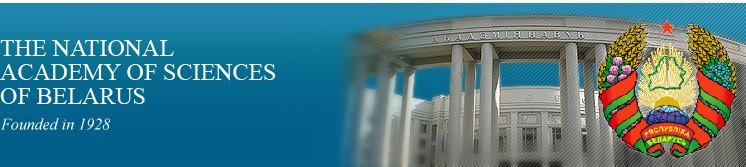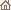Home News About Academy Academy Activities Academy Membership Organizations Publications Conferences Contacts Site Map

# Proc. of the Natl. Academy of Sciences of Belarus, Ser. Phys.-Math. Sci., 2001, No.4/ Publications / Scientific Journals

Proc. of the Natl. Academy of Sciences of Belarus, Ser. Phys.-Math. Sci., 2001, No.4Proceedings of the National Academy of Sciences of Belarus (Vestsi Natsiyanalnai Akademii Navuk Belarusi) SERIES OF PHYSICAL-MATHEMATICAL SCIENCESPublished by The Institute of Mathematics of NASB, Minsk, Republic of Belarus

### Number 4, 2001## CONTENTS

### MATHEMATICS

Silaeva N. A.
On the analog of the theorem of Schmidt in the 3-dimensional p-adic space. pp. 5--10

Summary: An extremality of general type curves in Q3p is proved.

Gribovskaya E. E.
Finite soluble groups with indices of maximal subgroups are p, p2 or 8. pp. 11--14

Summary: Let G be a finite soluble group whose index of every maximal subgroup is either a prime or a prime's square or 8. It is proved that p-chief rang rp(G/F(G)) £ 2 for every p > 2 and r2(G/F(G)) £ 3 and G Î N2'N2 U. From this result follows that a nilpotent length of a finite soluble group G is not more 4 and its p-length is not more 1 for every prime p > 2 and 2-length is not more 2.

Pal'chik E. M., Shmidt A. M.
On the finite groups admitting a coprime automorfism. pp. 15--18

Summary: The following main result is proved. Let X be a finite solvable group admitting automorhism y of prime order r, (|x|,r) = 1, B = Cx(y). If B a Hall subgroup of X then X = BF(X).

Benediktovitch I.I.
A free resolution of the Veronese's curve. pp. 19--24

Summary: Free resolution of a homogeneous ideal J (the ideal of the Veronese's curve) in a projective space Pn is calculated. The Grobner basis technique is used. Cohomologies of a bundle, associated with J, has been calculated.

Egorov A. D., Zherelo A. V.
About some questions of integration with respect to Gaussian mesuares on L2(X, g) space. pp. 25--30

Summary: A class of integrals with respect to Gaussian measures on L2(X,g) (where X is a linear topological space, g is Gaussian measure on X) which associates with quasimesures µ on X with characteristic functional of the form cµ(x) = exp {(-1)p/(2p)! K2p (x, ...(2p).., x)} is considered. For special cases of spaces X and measures µ^ orthonormal systems in reproducing Hilbert space of measure µ^ and approximation formulas for functional integrals with respect to measure µ^ are constructed.

Simonov A. Yu.
On optimality conditions for singular solutions of the general vector optimization problem. pp. 31--34

Summary: The problem of minimizing a mapping F: X® Y acting from a finite-dimensional normed space X into an ordered finite-dimensional vector space (Y, £) is considered. Under the assumption that the codimension of the positive cone of Y is equal to one high order necessary minimality conditions for singular (nonregular) points of F are proved.

Demenchuk A. K.
On almost periodic partially irregular solutions of ordinary differential systems. pp. 35--38

Summary: Let x. = A(t)x + X(t, x) be a almost periodic differential systems. Suppose that mod (A) Ç mod (X) = {0}. The existence problem for almost periodic irregular with respect to mod (A) solutions of such system is considered. This problem is reduced to the similar problem for a system of smaller dimension, and the sufficient conditions for existence of such solutions are obtained.

Zelenyak D. M.
The solving of dissipative inequalities for relaxation systems of second order. pp. 39--44

Summary: The set of solutions of dissipation inequality for relaxation systems of second order with direct input-output action is described. The extremal elements in this set are pointed out,

Rybachenko I. V.
About the estimation of deviation of rational interpolating operator of Fejer's type in limited interval. pp. 45--49

Summary: The estimation of deviation of special rational interpolating operator in uniform metrics for Lipschitz's functions of bounded variation was found. This estimation is less than best polynomial approximation in order sense.

Shilin A. P.
On the one boundary-value problem for two pairs of functions on the real axis. pp. 50--53

Summary: The boundary-value problem for two pairs of functions on the real axis in the case of rational coefficients is solved. The problem has the character of the Carleman boundary-value problem.

Zayats G. M.
On one problem about longitudinal impact on a composite elastic rod. pp. 54--57

Summary: The problem on longitudinal oscillations of a finite composite elastic rod has been investigated in the case when one of its ends is subjected to impact by some load which after a while disjoins from the rod.

Kurbachev A. V.
The calculation of vibrations transfer through a thin plate in solid elastic medium. pp. 58--61

Summary: The problem for the vibroisolating screen in the form of a thin plate placed in the elastic isotropic semi-space and effected by longitudinal and transversal waves has been considered. Taking into account wave energy losses in the elastic medium wave fields have been defined and the conditions under which the plate is the effective vibroisolating construction have been obtained.

### PHYSICS

Dorozhkin N. N., Ratkevich S. V.
Modified linear augmented-Slater-type-orbital method for electronic structure calculations of solids with arbitrary basis. pp. 62--67

Summary: The modified linear augmented Slater-type orbital method (LASTO) for solving Schrodinger's equation in crystals with arbitrary atoms per unit cell is presented. This method will allow more efficiently calculating an electronic band structure and structural energy difference for vary transition metal compounds. The analytic expressions for the overlap matrix and Hamiltonian matrix elements were obtained.

Makhnach V. V.
Bounds for the anapole interaction of the charged fermions at energy ~ 66 GeV. pp. 68--72

Summary: Analytical equations for the cross section and the forward-back asymmetry for reaction e- e+ ® l- l+ (l = µ, t) with respect to the anapole interaction are obtained. Bounds on numerical value of the anapole interaction parameter fore e-e+ ® µ-µ+ reaction at (ÖS) ~ 66 GeV are given.

Tikhomirov V. V., Yuralevich S. E.
Non-relativistic fermi-gas accretion onto a mini black hole. pp. 73--78

Summary: Matter accretion onto a mini-black hole (MBH) formed at an early stage of the Universe expansion is considered. The equations of a spherically symmetric adiabatic accretion of a relativistic Pascal liquid are derived. A simple expression for a mass absorption rate of non-relativistic Fermi gas by a MBH is obtained. A typical time of absorption of considerable amounts of the Earth's and neutron star's mass by a MBH is estimated. These estimates do not exclude the MBH presence in the Earth's depth and confirm the possibility of neutron star ignition by a captured MBH.

Petrova E. S.
Bessel light beams in magnetic gyrotropic media. pp. 79--83

Summary: Bessel light beams in magnetic gyrotropic media are investigated, The solution of the Maxwell's wave equations are obtained and polarization states for common case of arbitrary order vectorial Bessel beams are founded. The influence of gyrotropy on polarization state is discussed. The reflection and refraction of bessel beams on the boundary of gyrotropic media is calculated. The features of gyrotropy influence on reflection and refraction coefficients and polarization of the reflected and transmitted beams is also analyzed.

Khilo P. A.
Sum frequency generation by Bessel light beams under conditions of quasi-synchronism. pp. 84--89

Summary: Peculiarities of sum frequency generation by Bessel light beams when quasi-synchronous takes places in periodically polarized nonlinear crystals have been investigated.

Karpenko V. A., Romanenko A. A.
On possibility of determination of parameters of nanolayers by the modified Kretchman's sheme. pp. 90--92

Summary: It is shown that the modification of Kretchman's sheme by deposition of dielectric thin film on the metal one allows to increase sensitivity of the device to adsorbed layers. An analytical solution of the inverse problem of adsorbed layer parameters determination is obtained.

Chernichenko Yu. D.
Mathematical model of heating plate process by means of impulse laser radiation. pp. 93--95

Summary: In the plate the distribution of temperature is obtained by its the heating of impulse laser radiation with the space-time density distribution of absorbed stream light.

Smolik Ch. K. †, Makovetskii G. I., Severin G. M.
Determination of gold phonon density states function from the temperature dependence of specific heat. pp. 96--98

Summary: Using of the extrapolation method for solution of ill-posed problem described by the first kind Fredholm integer equation is considered. The method is used for calculating the phonon spectrum of gold from the temperature dependence of the specific heat. The minimal errors of the solution is achieved for operator norm ||A|| = 1.

Sukhvalo S. V., Vlasov V. V.
Temperature dependencies of the magnetic characteristics and factors of an anisotropy of films of alloys FeNiCo doped Ti and Si. pp. 99--102

Summary: The features of temperature changes of the magnetic characteristics of films of an alloy 14%Fe56%Ni30%Co doped Ti and Si in a range of temperatures 290--650 K are considered. The spin-reorientation transformations close 400 K and 550 K, accompanying by abnormal behaviour of coercive force and field of an anisotropy are revealed.

Karolik A. S.
Calculation of the dislocation electrical characteristics for one-valent metals. pp. 103--107

Summary: Within the framework of the partial-wave method the residual resistivity and thermoelectric characteristics of dislocations in noble (Cu, Ag, Au) and alkaline (K, Rb) metals are calculated. The quite satisfactory agreement with experiment is obtained. It is shown, that the thermoelectric power reveals by an order of magnitude higher sensitivity to the resonance level location relative to the Fermi energy in comparison with the residual resistivity.

Gaiduk P. I., Larsen A. N.
Rapid thermal annealing of arsenic-implanted Si1-xGex alloy layers: dose dependence. pp. 108--112

Summary: The structural defect evolution and dopant redistribution in arsenic-implanted relaxed Si1-xGex alloy layers have been studied by TEM and differential Hall measurements. The appearance of the particularities in the dopant profiles in the area of EOR-defect band is registered in Si1-xGex alloy layers implanted with arsenic at an average dose. This is found to correlate well with enhanced shrinkage of interstitial dislocation loops. The above features are connected to the formation of mobile dopant-vacancy complexes followed by complex-dislocation interaction.

### INFORMATICS

Klimovich C. G, Tikhonenko O. M.
Generalized characteristics of busy period distribution for a single server queueing system. pp. 113--117

Summary: The sum of tags random vector characteristics for demands served during busy period for queue are determined, taking into consideration the dependence between tags values and service time of the demand.

Malinkovsky Yu. V., Kravchenko S. V.
Queuing networks with "negative" requests, with the bypassing of request nodes and bounded queuing time. pp. 118--122

Summary: Open queuing networks with negative and positive requests, with the bypassing of request nodes and bounded queuing time is analyzed. It is ascertained that the stationary distribution of process states has a product form.

Astakhov A. M., Matalytskii M. A.
About finding of steady distribution for Markov queueing networks with many servers systems and different classes of customers. pp. 123--128

Summary: Queueing networks with many servers systems and different classes of customers have been considered. It is shown that transient probabilities depend on network states.

### SHORT NOTES

Tishchenko K. I.
On a problem of approximation of p-adic numbers by algebraic numbers. pp. 129--130

### ABSTRACTS OF DEPOSITED PAPERS

Bakhtin V. I.
Foliated functions and an operator of the averaged weighted shift for perturbations of hyperbolic maps. pp. 131

Bakhtin V. I.
Cramer's asymptotics in the averaging principle for systems with fast hyperbolic motions. pp. 131--132

Severenchuk N. B.
Semi-Browder essential spectra of linear operators in Banach space. pp. 132

Monastyrny P. I., Kuzhel'naya A. I.
On some selection procedures of control parameters and optimization of the marching algorithm and the reduction method. pp. 133

Akinfina M. A.
A consistent estimation of spectral density of a stationary stable casual process. pp. 133--134

In the Belarusian Physical Society. pp. 135--137

### SCIENTISTS OF BELARUS

Faina Mikhailovna Kirillova (On the occasion of the 70th birthday). pp. 138--139
Click
here to read this article in Russian (Windows 1251)/ Publications / Scientific Journals / Back to Top of Page

Designed and maintained by Dr. Nikolai N. Kostyukovich. Last updated: January 13, 2002
Created with assistance of Dr. Ignatii I. Korsak
Copyright © 2001, 2002 The National Academy of Sciences of Belarus
Copyright © 2001 The Institute of Mathematics of NASB